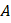# Which of the following statements are incorrect for the internal pressure of a van der Waals’ gas? a) It is independent of molar volume b) It is directly proportional to molar volume c) It is directly proportional to square of molar volume d) It is inversely proportional to square of molar volume

## Question ID - 102788 :- Which of the following statements are incorrect for the internal pressure of a van der Waals’ gas? a) It is independent of molar volume b) It is directly proportional to molar volume c) It is directly proportional to square of molar volume d) It is inversely proportional to square of molar volume

3537

 (a,b,c) From van der Waals’ equationInternal pressure is due to van der Waals’ forceFor two gasesandwith molecular weightsand, respectively, it is observed that at a certain temperature, the mean velocity ofis equal to theof. Thus, the mean velocity ofcan be made equal to the mean velocity of, if a)is at temperatureandis atsuch thatb) Temperature ofis lowered towhileis atsuch thatc) Bothandare raised to a higher temperature d) Heat energy supplied to